• Integer or Finite Decimal

• If your answer is an integer, for example, 23, enter 23. Do not enter 23.0.
• If your answer is a positive integer, don't enter the '+' sign. For example, if your answer is 23, enter 23, not +23.
• If your answer is a negative integer, please enter the '-' sign first. And there is no space between the '-' sign and the following digit. For example, enter '-23', not '- 23'.
• If for example your answer is 136.4, do not enter 136.40.
• No space or comma within an integer. For example, if your answer is 1000000. Please don't enter 1,000,000 or 1 000 000. Please enter 1000000.
• Fraction

• The numerator and denominator have to be positive integers. The numerator has to be less than the denominator. They can't have any common divisor.
• If your answer is a mixed fraction, i.e. an integer together with a proper fraction (the numerator being less than the denominator), such as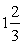, enter 1 in the leftmost part, 2 in the numerator part, and 3 in the denominator part.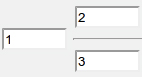If your answer is, enter 1 in the leftmost part (do not enter +1), 2 in the numerator part, and 3 in the denominator part.• If your answer is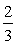, do not enter 0 in the leftmost part, leaving it blank. Enter 2 in the numerator part, and 3 in the denominator part.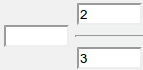• If your answer is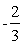, enter the minus sign, '-', in the leftmost part, 2 in the numerator part, and 3 in the denominator part.• If your answer is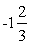, enter -1 in the leftmost part, 2 in the numerator part, and 3 in the denominator part.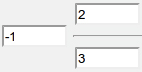• Repeating Decimal

• For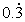, please enter• For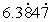, please enter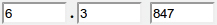• For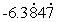, please enter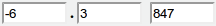• If your answer is 0.5, you can enter either 0.5 or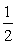. Any other answer is incorrect.
• If your answer is, you can enter eitheror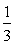. Any other answer is incorrect.
• If your answer is 9.2, you can enter either 9.2 or. Any other answer is incorrect.
• If your answer is 4.37, you can enter either 4.37 or. Any other answer is incorrect.